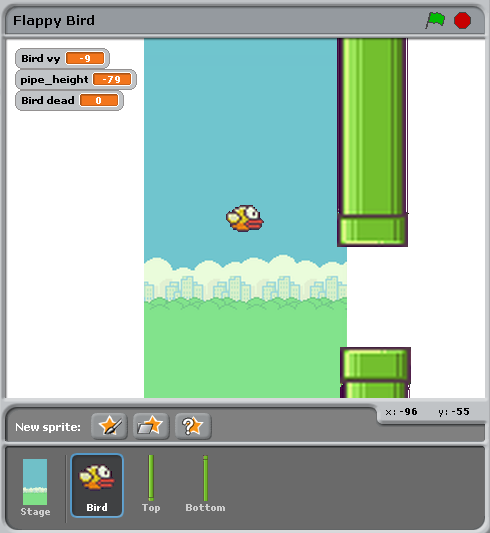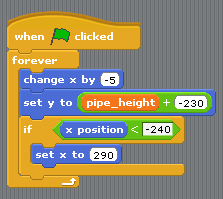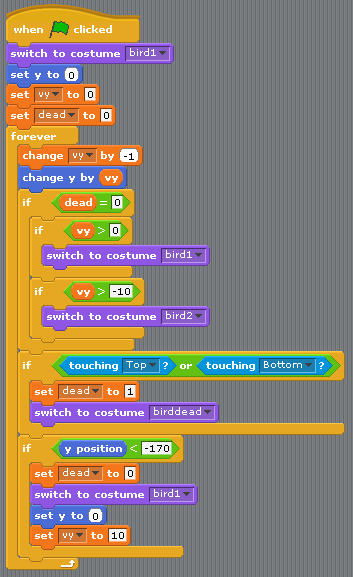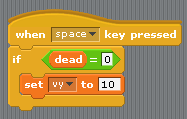# 从Scratch到Pygame Zero¶

Pygame Zero版本的游戏包含了积分逻辑，但是为了更清晰对比二者实现，所以在这份教程里忽略了积分。

## 舞台¶```bird = Actor('bird1', (75, 200))
pipe_top = Actor('top', anchor=('left', 'bottom'))
pipe_bottom = Actor('bottom', anchor=('left', 'top'))
```

```def draw():
screen.blit('background', (0, 0))
pipe_top.draw()
pipe_bottom.draw()
bird.draw()
```

## 管道移动¶• 当管道在屏幕左边即将出现的时候,条件 x position < -240 成立,并且会导致管道位置重置
• 变量 pipe_height 用来改变管道在数值方向的位置.上下管道之间的间隔应该是不变的,所以 不能让两个管道的位置都是随机的,所以我们只在一个脚本设置了让pipe_height随机的逻辑.
• 脚本 `set y position to pipe height +/- 230` 让顶部的管道在 `pipe_height` 上方 底部的管道在 `pipe_height` 下方.

```import random

WIDTH = 400
HEIGHT = 708
GAP = 130
SPEED = 3

def reset_pipes():
pipe_gap_y = random.randint(200, HEIGHT - 200)
pipe_top.pos = (WIDTH, pipe_gap_y - GAP // 2)
pipe_bottom.pos = (WIDTH, pipe_gap_y + GAP // 2)

def update_pipes():
pipe_top.left -= SPEED
pipe_bottom.left -= SPEED
if pipe_top.right < 0:
reset_pipes()
```

```def update():
update_walls()
```

## 小鸟¶

```GRAVITY = 0.3

# 初始化小鸟的状态
bird.vy = 0

def update_bird():
uy = bird.vy
bird.vy += GRAVITY
bird.y += bird.vy
bird.x = 75
```

• GRAVITY 指的的是 重力加速度 常数
• acceleration 用来改变 速度
• 速度用来改变小鸟的 位置

• 重力是向下的加速度常量: `GRAVITY` 大于0
• 加速度用来改变速度: 把 `GRAVITY` 加到 `bird.vy` 也就是小鸟竖直方向的速速
• 速度概念位置: 把 `bird.vy` 加到 `bird.y`

The next section makes the bird flap its wings:: 然后我们让小鸟可以拍打自己的翅膀:

```if not bird.dead:
if bird.vy < -3:
bird.image = 'bird2'
else:
bird.image = 'bird1'
```

```if bird.colliderect(pipe_top) or bird.colliderect(pipe_bottom):
```

```if not 0 < bird.y < 720:
bird.y = 200
bird.vy = 0
reset_pipes()
```

Again, this needs to be called every frame, so we add it to `update()`:: 再次重申,以上过程每一帧都要调用,因此我们把这些操作放到 `update_date()` 函数:

```def update():
update_walls()
update_bird()
```

```FLAP_VELOCITY = -6.5

def on_key_down():
bird.vy = FLAP_VELOCITY
```Scratch和Pygame Zero最大的不同在于:

• 在Pygame Zero中你不能一直循环 - 只更新每一帧并且返回
• 坐标系不同.Pygame Zero,屏幕的左上角是原点 `x = 0, y = 0`, `x` 跟Scratch一样是 从左向右的,但是 `y` 轴指向屏幕下方! 这也是 `GRAVITY` 是正数而 `FLAP_VELOCITY` 是负数 了.
• `bird.dead` 是一个布尔值, 所以我们写 `if not bird.dead` 而不是像Scratch一样 写 `dead = 0`.

## 总结¶

Many of the concepts available in Scratch can be translated directly into Pygame Zero. 许多Scratch种的概念可以直接迁移到Pygame Zero中.

In Scratch In Pygame Zero
`change y by 1` (up) `bird.y -= 1`
`change y by -1` (down) `bird.y += 1`
`set costume to <name>` `bird.image = 'name'`
`if dead = 0` `if not bird.dead:`
`set dead to 0` `bird.dead = False`
`if touching Top?` `if bird.colliderect(pipe_top)`
`When Flag clicked``forever` Put code into the `update()` function.
`When [any] key pressed` `def on_key_down():`
`pick random a to b` `import random` to load the `random` module, then `random.randint(a, b)`
(0, 0) 是屏幕中心 (0, 0) 是屏幕的左上角

Pygame Zero中的角色使得我们更加方便的使用坐标系.我们使用 `anchor` 中心点位置来决定 管道的位置, 我们通过检测管道的 `pipe_top.right < 0` 而不是 `if x position < -240` 来判断关掉是否离开屏幕.